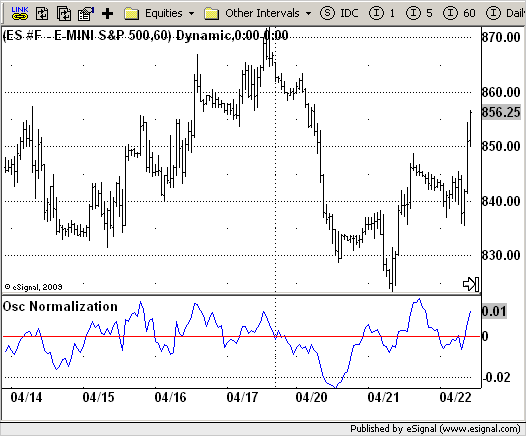# Osc Normalization

ICE Data Services -

Normaliz.efs

File Name: Normaliz.efs

Description:
Osc Normalization

Formula Parameters:

• NormType : 1
• OscLength : 10
• NormLength : 10
• Source of Normalizing : Close
• Source of Oscillator : Close

Notes:
This indicator is created for normalizing any other indicators using its functions.
The first function to be created is one based on Bell's technique for normalizing to average price. This function has three input values. OscValue represents the value of the oscillator to be normalized. This input is included in each of the four functions. The NormPrice and NormLength inputs represent the price and length values used to calculate the average that is used in the normalization.
The second function is for Bell's technique for normalizing to standard deviation of prices. This function also has three input values. I already described the OscValue input. The NormPrice and NormLength inputs represent the price and length values used to calculate the standard deviation that is used in the normalization.
The third function is for Bell's techniques for normalizing to average range of prices. Unlike the two previous functions, this function only has two inputs: the OscValue input, and the NormLength input, which represents the number of bars to use in the calculation of the average true range that is used in the normalization.
The fourth function is for Bell's technique for normalizing to range. This function also has only two inputs: the OscValue input, and a NormLength input, which represents the number of bars to use for the historical range that is used in the normalization.

Normaliz.efsEFS Code:

```/*********************************
Provided By:
eSignal (Copyright c eSignal), a division of Interactive Data
Formula Script (EFS) is for educational purposes only and may be
modified and saved under a new file name.  eSignal is not responsible
for the functionality once modified.  eSignal reserves the right
to modify and overwrite this EFS file with each new release.

Description:
Osc Normalization

Version:            1.0  04/22/2009

Formula Parameters:                     Default:
NormType                            1
OscLength                           10
NormLength                          10
Source of Normalizing               Close
Source of Oscillator                Close

Notes:
This indicator is created for normalizing any other indicators using its functions.
The first function to be created is one based on Bell's technique for
normalizing to average price. This function has three input values. OscValue
represents the value of the oscillator to be normalized. This input is included
in each of the four functions. The NormPrice and NormLength inputs represent the
price and length values used to calculate the average that is used in the normalization.
The second function is for Bell's technique for normalizing to standard deviation
of prices. This function also has three input values. I already described the OscValue
input. The NormPrice and NormLength inputs represent the price and length values used
to calculate the standard deviation that is used in the normalization.
The third function is for Bell's techniques for normalizing to average range of prices.
Unlike the two previous functions, this function only has two inputs: the OscValue input,
and the NormLength input, which represents the number of bars to use in the calculation of
the average true range that is used in the normalization.
The fourth function is for Bell's technique for normalizing to range. This function also
has only two inputs: the OscValue input, and a NormLength input, which represents the number
of bars to use for the historical range that is used in the normalization.

**********************************/
var fpArray = new Array();
var bInit = false;

function preMain() {
setStudyTitle("Osc Normalization");
setCursorLabelName("Norm Osc", 0);
setCursorLabelName("Balance", 1);
setDefaultBarFgColor(Color.blue, 0);
setDefaultBarFgColor(Color.red, 1);
var x = 0;
fpArray[x] = new FunctionParameter("NormType", FunctionParameter.STRING);
with(fpArray[x++]) {
setName("Type of Normalization");
setDefault("Average");
}
fpArray[x] = new FunctionParameter("OscLength", FunctionParameter.NUMBER);
with(fpArray[x++]) {
setName("Length of Oscillator");
setLowerLimit(1);
setDefault(10);
}
fpArray[x] = new FunctionParameter("NormLength", FunctionParameter.NUMBER);
with(fpArray[x++]) {
setName("Length of Normalization");
setLowerLimit(1);
setDefault(8);
}
fpArray[x] = new FunctionParameter("sNormPrice", FunctionParameter.STRING);
with(fpArray[x++]){
setName("Source of Normalization");
setDefault("close");
}
fpArray[x] = new FunctionParameter("sOscPrice", FunctionParameter.STRING);
with(fpArray[x++]){
setName("Source of Oscillator");
setDefault("close");
}
}

var xMOM = null;
var xHHMOM = null;
var xLLMOM = null;
var xMA = null;
var xPriceOsc = null;
var xPriceNorm = null;
var xNormStdDev = null;

function main(NormType, sOscPrice, OscLength, sNormPrice, NormLength) {
var nBarState = getBarState();
if (nBarState == BARSTATE_ALLBARS) {
if (NormType == null) NormType = "Average";
if (sOscPrice == null) sOscPrice = "close";
if (OscLength == null) OscLength = 10;
if (sNormPrice == null) sNormPrice = "close";
if (NormLength == null) NormLength = 8;
}
if (bInit == false) {
xPriceOsc = eval(sOscPrice)();
xPriceNorm = eval(sNormPrice)();
xMOM = mom(OscLength, xPriceOsc);
xHHMOM = upperDonchian(NormLength, xMOM);
xLLMOM = lowerDonchian(NormLength, xMOM);
xMA = sma(NormLength, xPriceNorm);
xNormStdDev = efsInternal("Calc_StdDev", NormLength, xPriceNorm, xMA)
xATR = atr(NormLength);
setCursorLabelName(NormType, 0);
if (NormType != "Range") {
} else {
}
bInit = true;
}
var OscValue = xMOM.getValue(0);
var AvgValue = xMA.getValue(0);
var NormAvg = 0;
if (AvgValue != 0) NormAvg = OscValue / AvgValue;
var StdDev = xNormStdDev.getValue(0);
var NormStdDev = 0;
if (StdDev != 0) NormStdDev = OscValue / StdDev;
var ATR = xATR.getValue(0);
var NormATR = 0;
if (ATR != 0) NormATR = OscValue / ATR;
var HiHi = xHHMOM.getValue(0);
var LoLo = xLLMOM.getValue(0);
if (HiHi == null) return;
var HighLowDiff = HiHi - LoLo;
var NormRange = 0;
if (HighLowDiff != 0) NormRange = (((OscValue - LoLo) / HighLowDiff) * 100);
var nRes = 0;
if (NormType == "Average") {
nRes = (NormAvg);
}
else if (NormType == "StdDev") {
nRes = (NormStdDev);
}
else if (NormType == "ATR") {
nRes = (NormATR);
}
else if (NormType == "Range") {
nRes = (NormRange);
}
return nRes;
}

function Calc_StdDev(Period, xSeries1, xSeries2) {
var nRes = 0;
var sum = 0;
var barsBack = 0;
var nSeries1 = xSeries1.getValue(0);
if (nSeries1 == null) return;
for (barsBack = 0; barsBack < Period; barsBack++) {
sum += (xSeries1.getValue( - barsBack) - xSeries2.getValue(0)) * (xSeries1.getValue( - barsBack) - xSeries2.getValue(0));
}
nRes = Math.sqrt(sum / Period);
return nRes;
}```